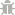Light Mode
Rate us!# area converter

To use the area converter, click the area converter from the menu on the left and write the value you want to convert to other units of areas in the “From” field, and select the area unit in which you want to transform that value from the “To” field. You will be able to see the results right after you click on your desired unit of conversion.

From: Acre (acre)
To: Acre (acre)

## The Area Calculator

As you already know that area is the measurement of the amount of space on a flat surface. For example, one yard is equal to three feet, and two yards will have twice the area of one yard because there is twice as much space in one. Now, when it comes to finding an area of the land, all the shapes have different ways of finding their area. As in a rectangular surface if we want to find space manually, then we will use the formula based on pure mathematics which is width times the length.
Also, an area is usually measured in square units where a unit can be any standard magnitude of a quantity, and there is one standard unit of the area known as the square meter which can be used in any rectangular or square shape. A square meter is defined as the area of a square with each of its side equal to one meter and is typically used to measure the areas of lands. A square meter is either utilized for describing small areas whereas acre and a arectare is used for properties with more significant lengths and widths.
There are almost four thousand square meters in one acre, hundred square meters in one are, and ten thousand square meters in one hectare. For exact calculations use our area calculator or call it are a converter or land measurement calculator which can be used to find any area of the square.

## Area Converter And Calculator For Flooring

By converting the area to square feet, you can quickly learn about how much material your room, project, office, etc. will require. You can use our other calculators to find the exact cost of equipment which will be utilized for your project. If we talk about manually checking the size of the area, it will take a lot of time as you will need to use an inches tape or a metering rod for checking meter squares. You won’t know if your result is accurate after making calculations because precise calculations are hard to perform manually.

### How To Convert Area With Online Square FootTransparent

Our tool has a lot of area units ready to make your calculations. Finding areas not only helps in checking how much space a building etc. would acquire but also can be used in solving mathematical problems for students. You can use the area calculator to convert inches to meters, and can also check an area of a circle.

To use this area of a square calculator all you have to do is check the steps down below.

1. The first thing you will see on the page is a Unit Converter with a lot of tools in its menu, after tapping on area calculator you can add values that you wish to change in its first column that looks like this:
2. After adding value, you can choose the required forms, from the menus below the field, let’s say you want to change a square kilometer to square meters you will have to select the square kilometer from the first menu and square meter from the second menu like this:
3. The answer will be displayed in the “TO” field as shown in the above picture.

### AS SEEN ON:

Plagiarism Checker